Open in App
Not now

# Puzzle | Three Squares

• Difficulty Level : Easy
• Last Updated : 18 Jan, 2023

Given four sticks of length ‘a’ and four more sticks of length ‘b’. The length of ‘b’ is exactly half of the length of ‘a’. The task is to make three equal squares using the given 8 sticks.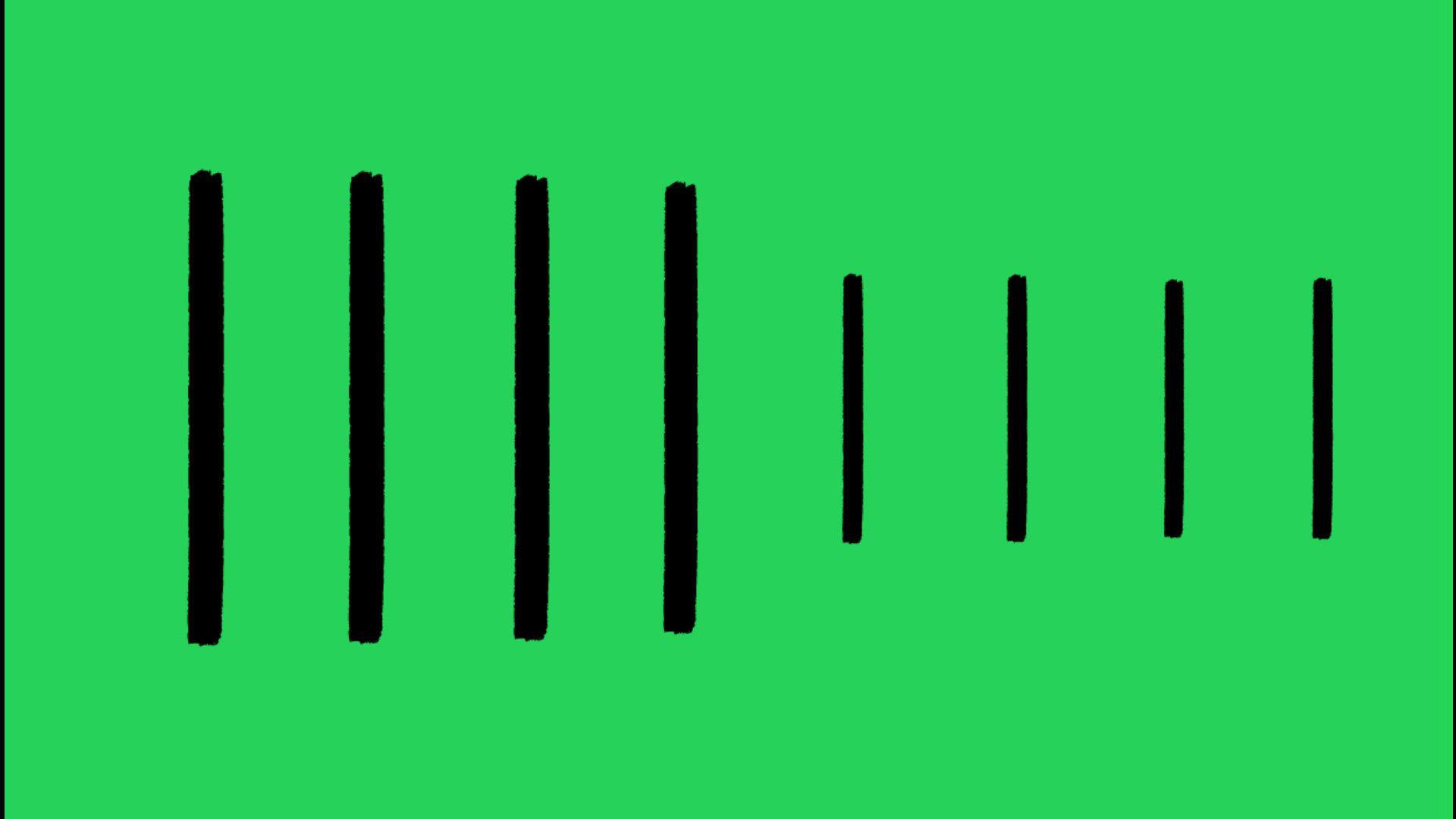Solution:
Below are the steps to make three equal squares from the given sticks:

1. Place the first stick of length a horizontally.2. Now place the second stick of length a vertically such that it cuts the previously placed stick into two equal halves.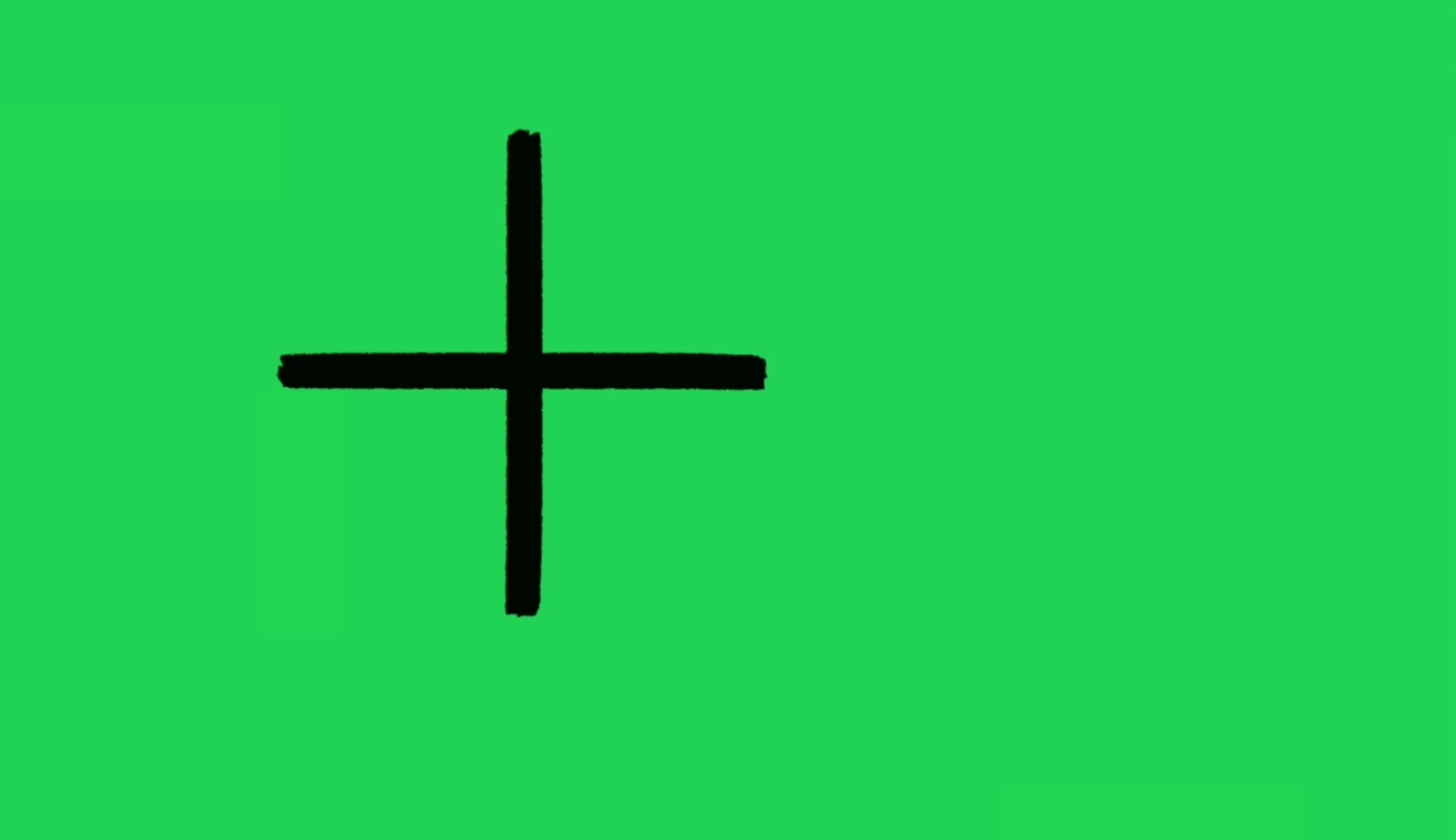3. Place the third stick of length a horizontally connecting the bottom point of the vertically placed stick in last steps.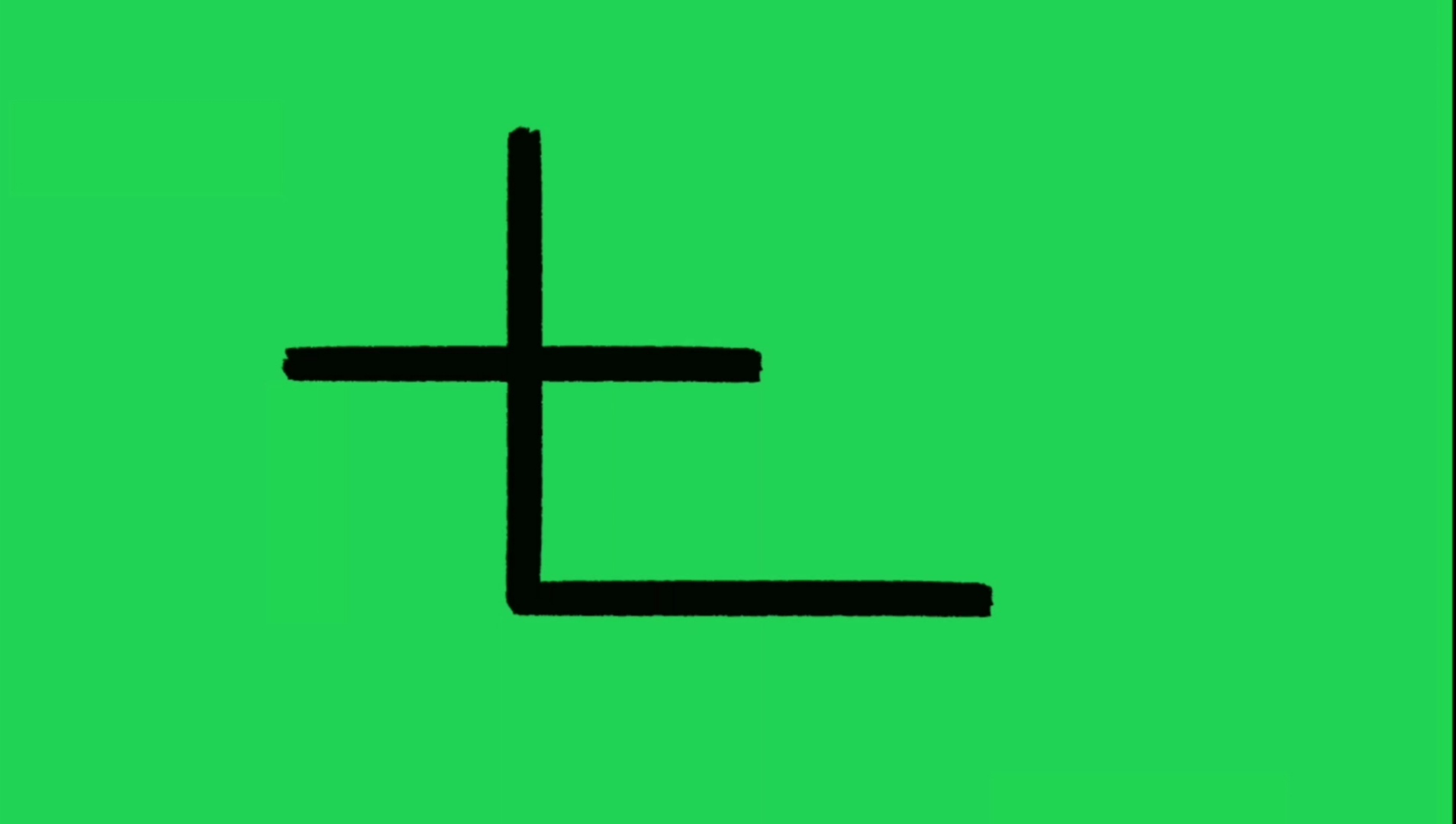4. Now, place the forth stick of length a vertically such that it cuts the last placed stick into two halves and a square of size a/2 ( = b ) is formed.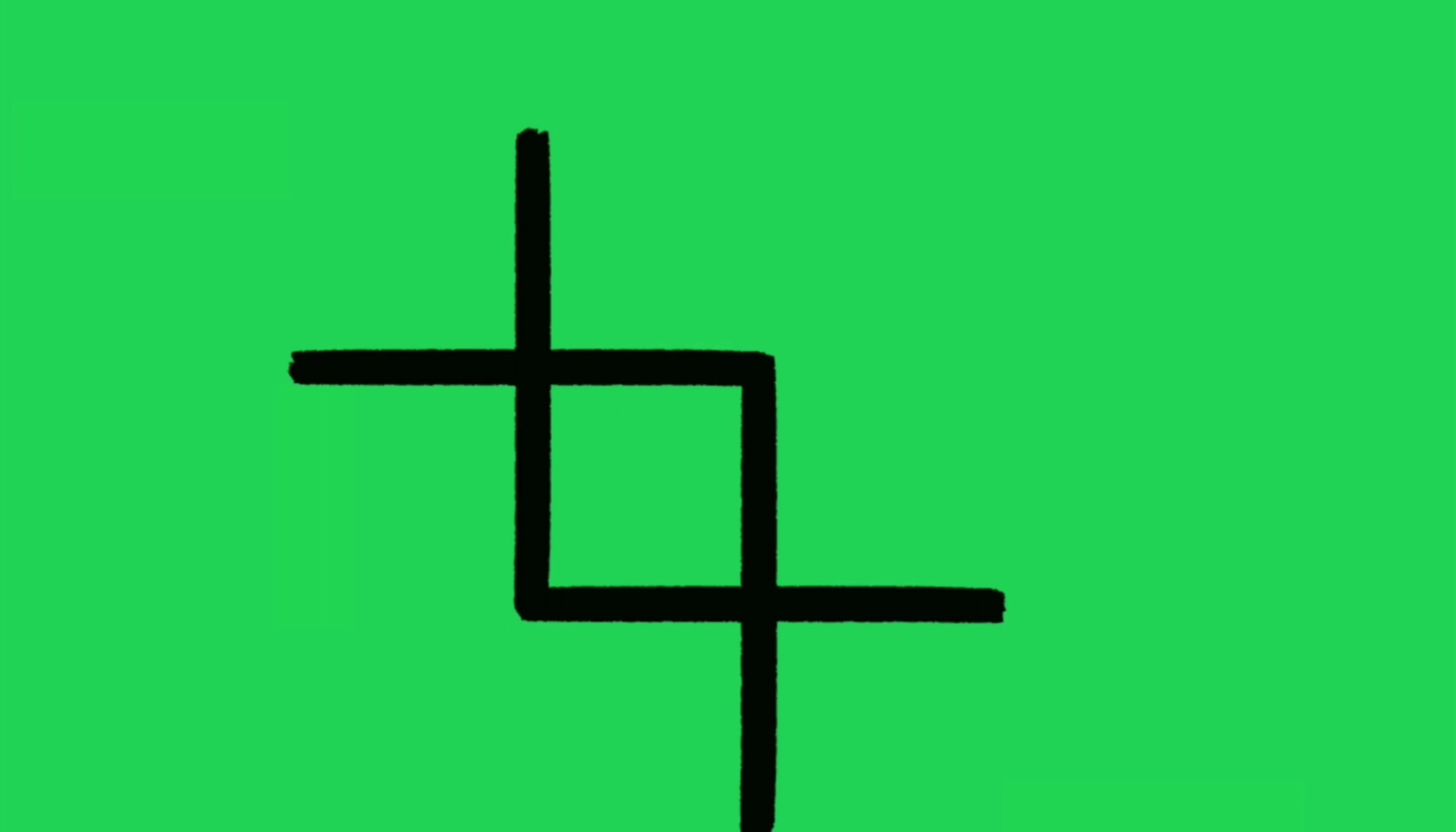5. Now, only 4 sticks of length b are left and 2 more squares are left to be formed. Use the remaining 4 sticks at the corners of the remaining sides of squares of side a/2 ( = b) .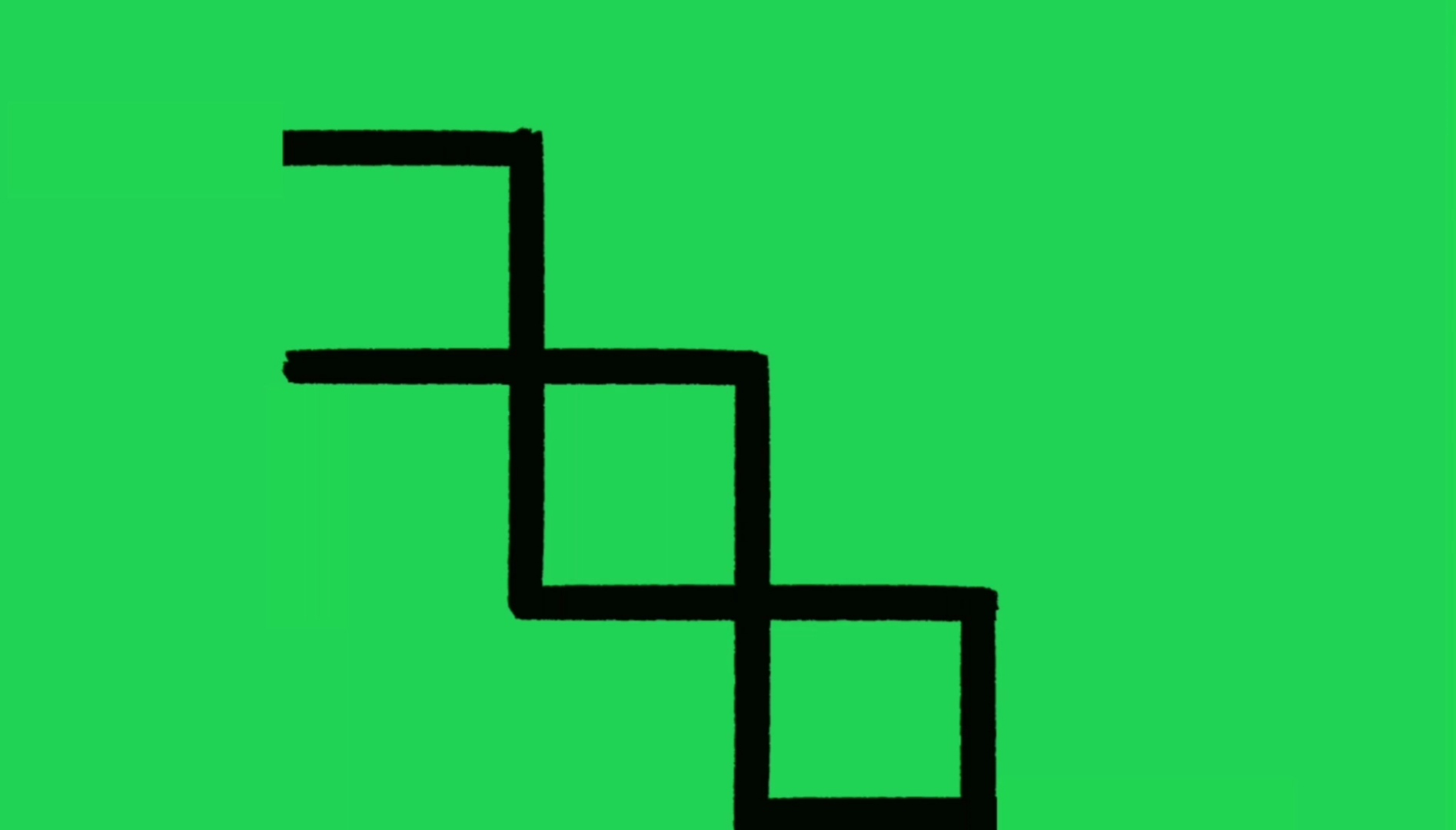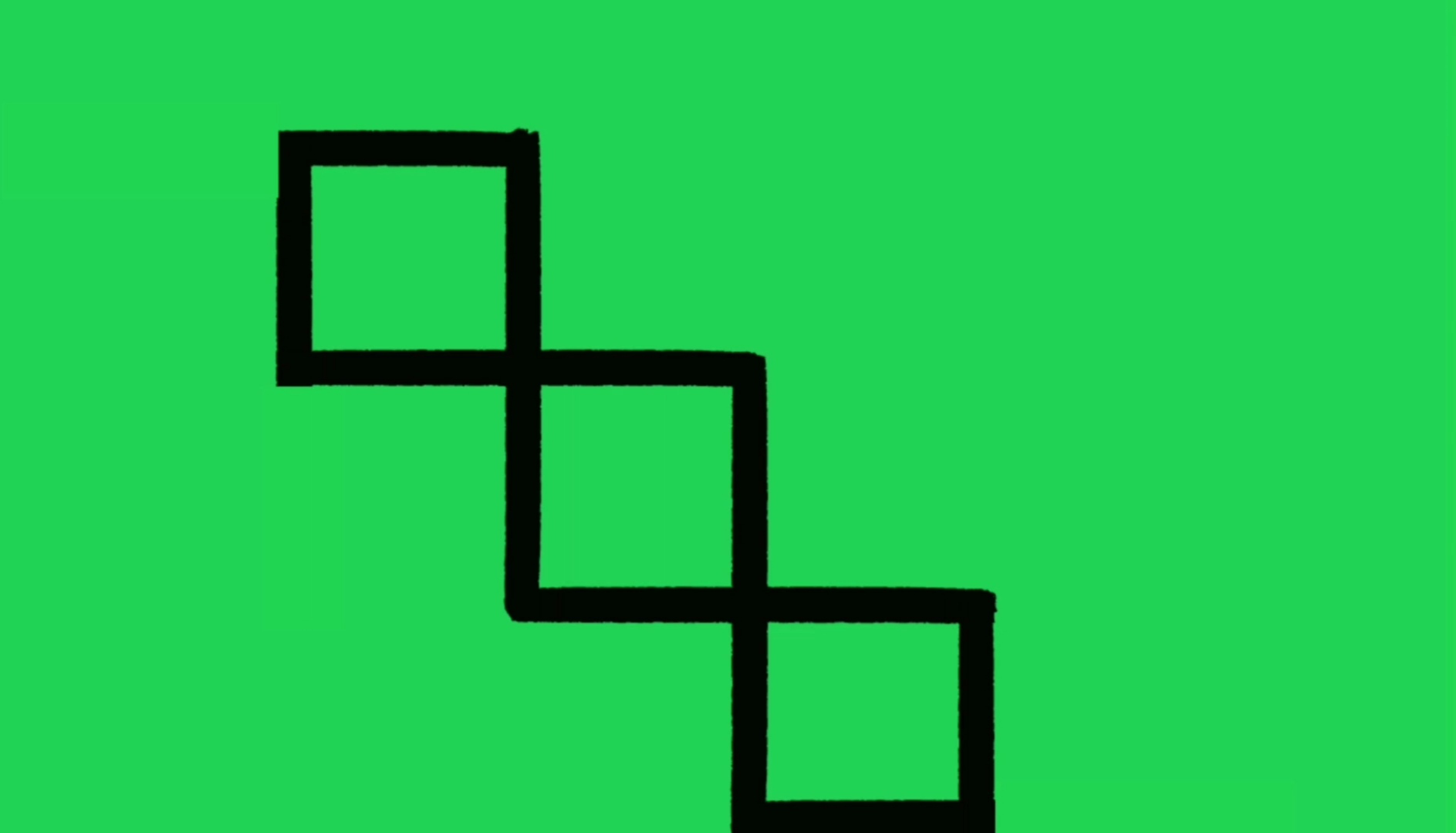Below is the video of the above illustration:

My Personal Notes arrow_drop_up